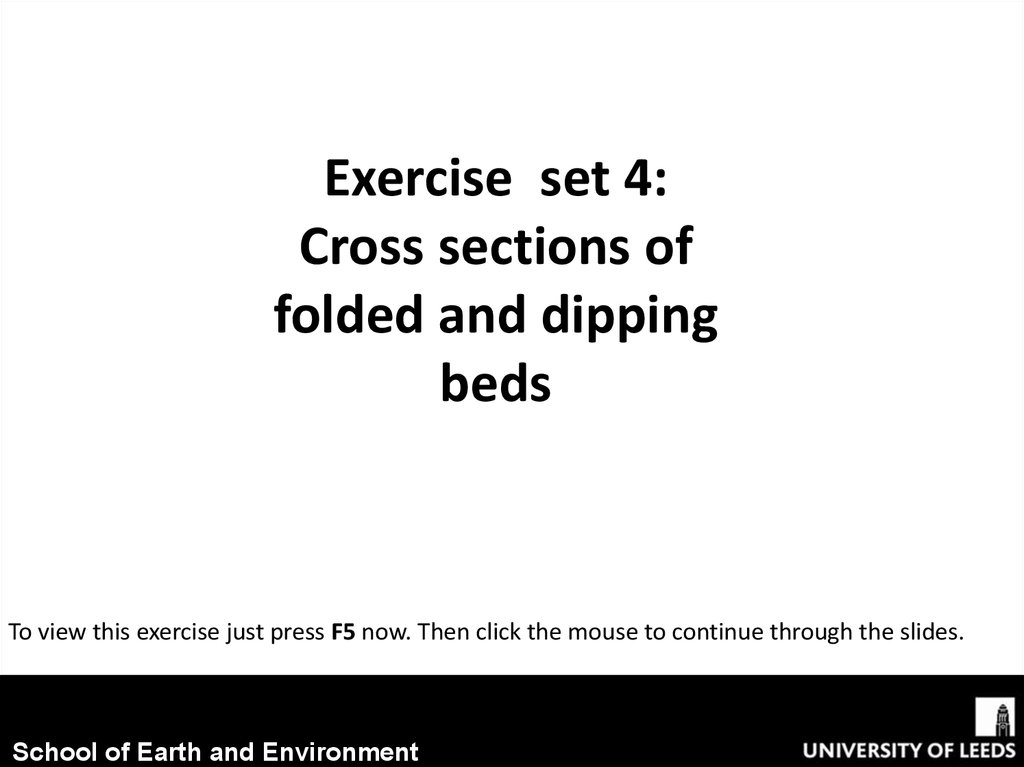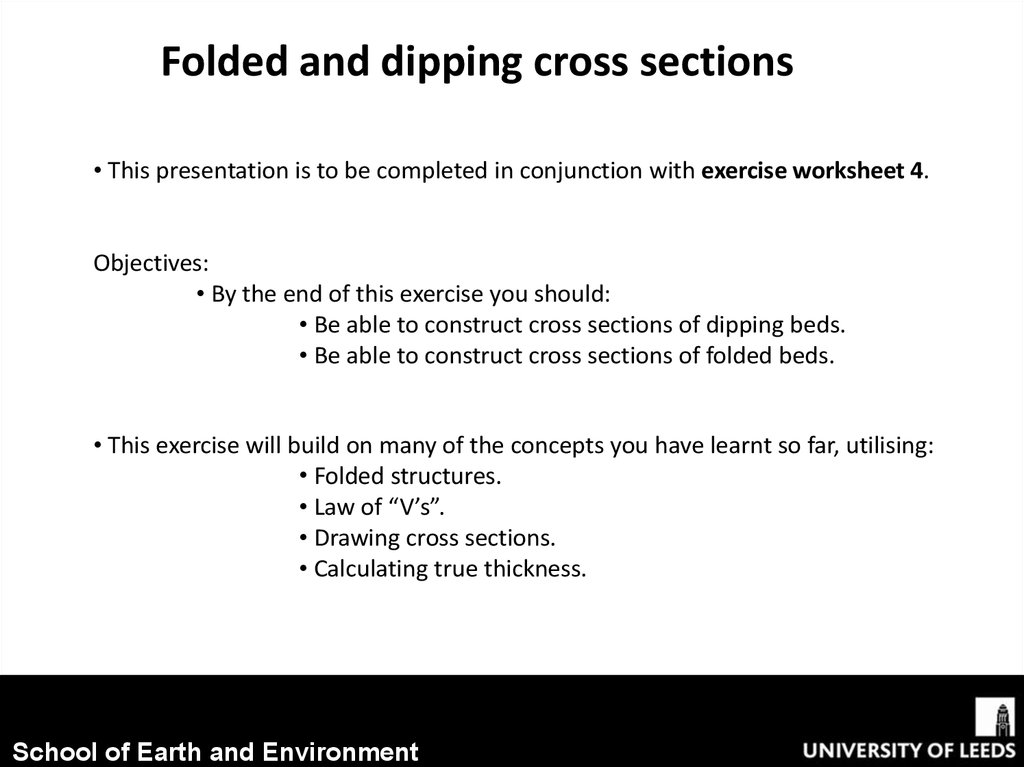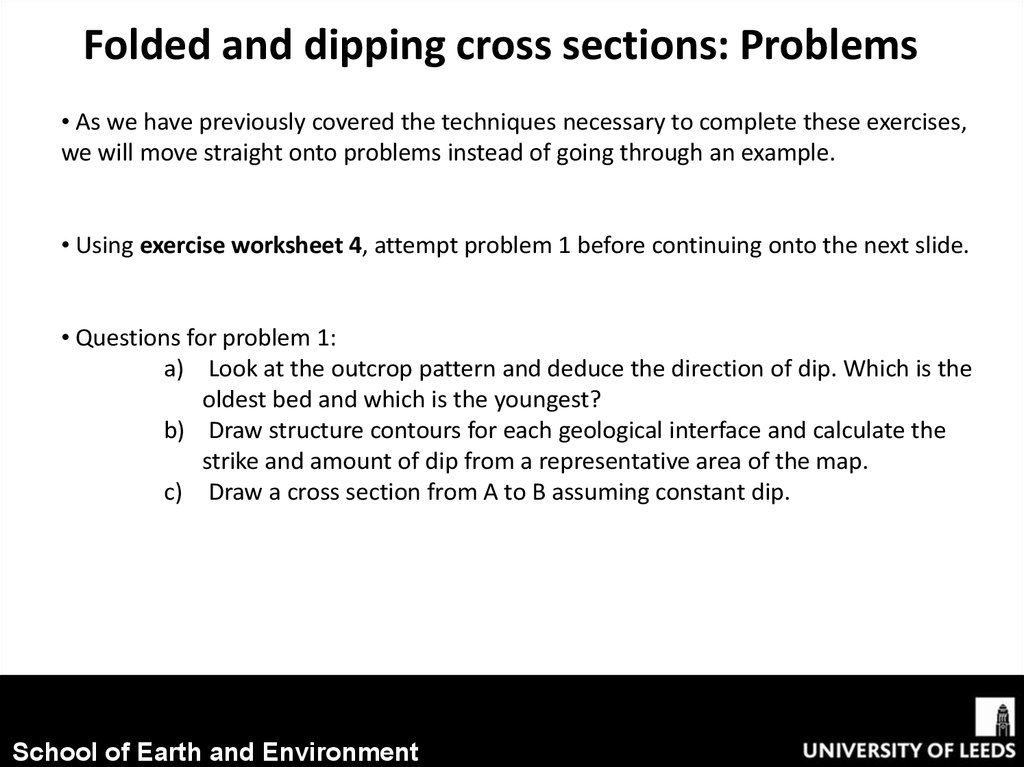# Cross sections of folded and dipping beds

## 1.

Exercise set 4:
Cross sections of
folded and dipping
beds
To view this exercise just press F5 now. Then click the mouse to continue through the slides.
School of Earth and Environment

## 2.

Folded and dipping cross sections
• This presentation is to be completed in conjunction with exercise worksheet 4.
Objectives:
• By the end of this exercise you should:
• Be able to construct cross sections of dipping beds.
• Be able to construct cross sections of folded beds.
• This exercise will build on many of the concepts you have learnt so far, utilising:
• Folded structures.
• Law of “V’s”.
• Drawing cross sections.
• Calculating true thickness.
School of Earth and Environment

## 3.

Folded and dipping cross sections: Problems
• As we have previously covered the techniques necessary to complete these exercises,
we will move straight onto problems instead of going through an example.
• Using exercise worksheet 4, attempt problem 1 before continuing onto the next slide.
• Questions for problem 1:
a) Look at the outcrop pattern and deduce the direction of dip. Which is the
oldest bed and which is the youngest?
b) Draw structure contours for each geological interface and calculate the
strike and amount of dip from a representative area of the map.
c) Draw a cross section from A to B assuming constant dip.
School of Earth and Environment

## 4.

Folded and dipping cross sections: Problems
a) Following the law of “V’s” that: Beds dipping
downstream V-downstream ; the beds must dip to
the South.
Then following the law of superposition stating that:
“In any un-overturned sequence of rocks deposited in
layers, the youngest layer is on top and the oldest on
the bottom; each layer being younger than the one
beneath it and older than the one above”
Then the series of deposition must be:

## 5.

Folded and dipping cross sections: Problems
b) Remember: to draw structure contours, the
geological boundary is known where it
crosses a topographic contour line. For
example a line can be drawn through the
four geological boundaries where they cross
the 600m contour.
Then use a protractor to measure strike;
Strike = 85o
School of Earth and Environment

## 6.

Folded and dipping cross sections: Problems
b) Then to calculate dip, choose two representative structure contours, eg. 400m and
500m. Using your ruler measure the distance between these on the map (250m),
this gives you the adjacent length of the triangle (see below). Then calculate the
difference in height from the structure contours (100m) , this gives you the opposite
side of the triangle.
Then using simple trigonometry:
tan(θ) = (100m/250m)
tan-1(100m/250m) = θ = 22°
True dip = 22°
School of Earth and Environment

## 7.

Folded and dipping cross sections: Problems
c) First mark on the topographic points.
School of Earth and Environment

## 8.

Folded and dipping cross sections: Problems
c) Then mark on the geological interface points.
School of Earth and Environment

## 9.

Folded and dipping cross sections: Problems
c) Then using a protractor, measure a dip of 22o and draw on the dipping beds.
School of Earth and Environment

## 10.

Folded and dipping cross sections: Problems
c) Now fill in the beds lithological patterns.
School of Earth and Environment

## 11.

Folded and dipping cross sections: Problems
c) Finally extend the structure contours, to show where the beds would extend to if
School of Earth and Environment

## 12.

Folded and dipping cross sections: Problems
• Using exercise worksheet 4, complete problem 2 before continuing onto the next
slide.
• Questions for problem 2:
a) On the map draw structure contours for each boundary (i.e. SiltstoneShale boundary; Shale-Grit boundary).
b) Calculate the true thickness of the shale bed.
c) Using the topographic and structure contours, construct a cross section
through A to B.
d) Indicate on the map the position of an anticlinal axis with the symbol:
and a synclinal axis with the symbol:
School of Earth and Environment

## 13.

Folded and dipping cross sections: Problems
a) Remember: to draw
structure contours, the
geological boundary is
known where it crosses a
topographic contour line.
Blue = Siltstone-shale boundaries; Green = Grit-shale boundaries
School of Earth and Environment

## 14.

Folded and dipping cross sections: Problems
b) Remember to calculate true thickness:
True thickness (t) = width of outcrop (w) x sin(θ) (angle of dip)
So first we must calculate the angle of dip using structure contours (e.g. the most
Westerly shale structure contours: 700m and 600m.)
The distance between these is: ~9mm = 180m.
The difference in height is: 700m-600m = 100m
Therefore:
tan(θ) = (100m/180m)
tan-1(100m/180m) = θ = 29°
True dip = 29°
So:
True thickness (t) = 370m x sin(29°)
True thickness (t) = 179 m
School of Earth and Environment

## 15.

Folded and dipping cross sections: Problems
c) First mark on the topographic points.
School of Earth and Environment

## 16.

Folded and dipping cross sections: Problems
c) Then mark on the lithological interfaces.
School of Earth and Environment

## 17.

Folded and dipping cross sections: Problems
c) Then assuming constant dip, structure contour points from other areas of the map
School of Earth and Environment

## 18.

Folded and dipping cross sections: Problems
c) Now the beds can be drawn on. Remember to use solid lines where the actual
boundaries are and dotted lines for where the boundaries are unknown as well as
where they would of extended to above ground level prior to erosion.
How does the measured thickness of the cross section compare with your calculated
actual thickness?
School of Earth and Environment

## 19.

Folded and dipping cross sections: Problems